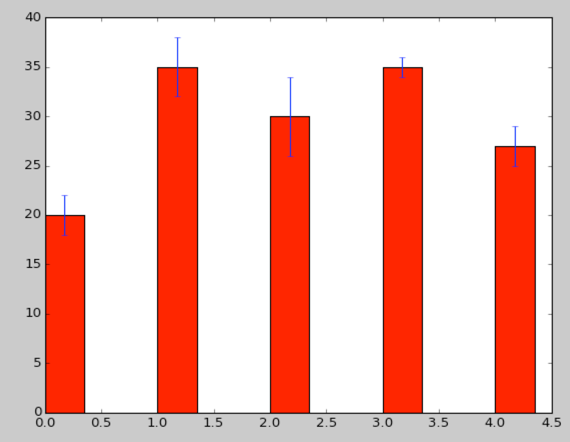# Python: matplotlib hangs and shows nothing (Mac OS X)

I've been playing around with some of the matplotlib demos recently and discovered that simply copying one of the examples didn't actually work for me.

I was following the bar chart example and had the following code:

``````
import numpy as np
import matplotlib.pyplot as plt

N = 5
ind = np.arange(N)
fig, ax = plt.subplots()
menMeans = (20, 35, 30, 35, 27)
menStd =   (2, 3, 4, 1, 2)
width = 0.35       # the width of the bars
rects1 = ax.bar(ind, menMeans, width, color='r', yerr=menStd)

plt.show()

``````

When I execute this script from the command line it just hangs and I don't see anything at all.

Via a combination of different blog posts (which all suggested different things!) I ended up with the following variation of imports which seems to do the job:

``````
import numpy as np
import matplotlib
matplotlib.use('TkAgg')
import matplotlib.pyplot as plt

N = 5
ind = np.arange(N)
fig, ax = plt.subplots()
menMeans = (20, 35, 30, 35, 27)
menStd =   (2, 3, 4, 1, 2)
width = 0.35       # the width of the bars
rects1 = ax.bar(ind, menMeans, width, color='r', yerr=menStd)

plt.show()

``````

If I run this script a Python window pops up and contains the following image which is what I expected to happen in the first place!The thing to notice is that we've had to change the backend in order to use matplotlib from the shell:

With the TkAgg backend, which uses the Tkinter user interface toolkit, you can use matplotlib from an arbitrary non-gui python shell.

Current state: Wishing for ggplot!